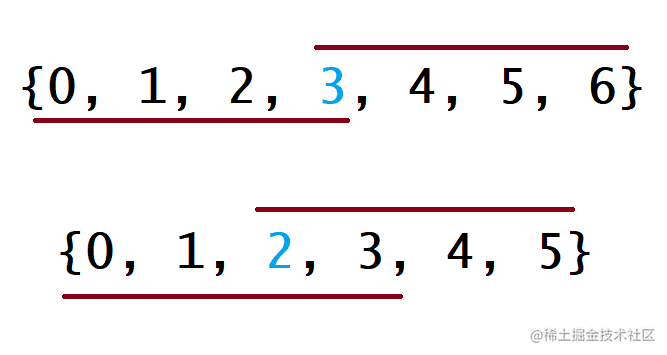# 题目

• 输入：nums = [3,2,3]
• 输出：3

• 输入：nums = [2,2,1,1,1,2,2]
• 输出：2

# 方法一：哈希表

## 代码

class Solution {
func majorityElement(_ nums: [Int]) -> Int {
var counts: [Int : Int] = [:]
var majority: Int = 0
for num in nums {
var cnt: Int = counts[num] ?? 0
cnt += 1
if cnt > (nums.count / 2) {
majority = num
break
}
counts[num] = cnt
}
return majority
}
}

## 复杂度分析

• 时间复杂度：时间复杂度：O(n)，其中 n 是数组 nums 的长度。我们遍历数组 nums 一次，对于 nums 中的每一个元素，将其插入哈希表都只需要常数时间。如果在遍历时没有维护最大值，在遍历结束后还需要对哈希表进行遍历，因为哈希表中占用的空间为 O(n)（可参考下文的空间复杂度分析），那么遍历的时间不会超过 O(n)。因此总时间复杂度为 O(n)。

• 空间复杂度：O(n)。哈希表最多包含 $n - \lfloor \dfrac{n}{2} \rfloor$ 个键值对，所以占用的空间为 O(n)。这是因为任意一个长度为 n 的数组最多只能包含 n 个不同的值，但题中保证 nums 一定有一个众数，会占用（最少）$\lfloor \dfrac{n}{2} \rfloor + 1$ 个数字。因此最多有 $n - (\lfloor \dfrac{n}{2} \rfloor + 1)$ 个不同的其他数字，所以最多有 $n - \lfloor \dfrac{n}{2} \rfloor$ 个不同的元素。

# 方法二：排序

## 思路及解法## 代码

class Solution {
func majorityElement(_ nums: [Int]) -> Int {
var tempNums = nums
tempNums.sort()
return tempNums[tempNums.count / 2]
}
}

## 复杂度分析

• 时间复杂度：O(n log n)。将数组排序的时间复杂度为 O(n log n)。

• 空间复杂度：O(logn)。如果使用语言自带的排序算法，需要使用 O(logn) 的栈空间。如果自己编写堆排序，则只需要使用 O(1) 的额外空间。

iOS